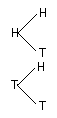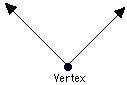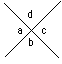Math Central - mathcentral.uregina.caResource Room— glossaries Resource RoomMathematics Glossary - Middle Years Maxine Stinka, Saskatchewan Education, Curriculum and Instruction Note: The definitions included here are those that are used in the Saskatchewan Education document "Mathematics 6-9: A Curriculum Guide for the Middle Level". Various mathematics dictionaries may have different definitions. These definitions are designed to be meaningful to middle level mathematics teachers. Select the first letter of the term that you wish to look for. A | B | C | D | E | F | H | I | J | K | L | M | N | O | P | Q | R | S | T | U | V | W | X | Y | Z T term any expression written as a product or quotient. e.g. 3xy, 2m3, or -5x3y2z tessellate the repeated use of geometric figures to completely cover a plane without overlapping. theoretical probability probability that is determined on the basis of reasoning, not through experimentation. e.g. Since a regular die has 6 sides, the theoretical probability of tossing a 3 is 1/6.   transformation a movement of one geometric shape to another according to some rule. The common transformations used in middle level mathematics are translations, and reflections. translation (slide) an exact duplication of a geometric figure formed by moving each point in the figure the same distance and in the same direction. a line that intersects 2 or more other lines in the same plane. a quadrilateral with at least one pair of parallel sides. tree diagram a diagram representing a systematic way of determining all possible outcomes in a probability experiment. e.g. if you toss two coins, the possible outcomes are (H,H), (H,T), (T,H) and (T,T):trinomial a polynomial consisting of 3 terms. turn see rotation. U unit price the price of a single item or the price per kilogram or gram. unlike terms terms with different variables or the same variables raised to different exponents. e.g. 4x2 and 2x3. V a symbol, usually a small case letter, used to represent one or more numbers. e.g. In the expression 2x + 3, the variable is x. The 3 is called a constant because its value never changes. vertex (plural: vertices) the point of intersection of two rays that form an angle, two sides of a polygon or two edges of a solid.vertically opposite angles two angles formed by the intersection of two lines. They share a common vertex but no sides or interior points. e.g.Vertically opposite angles, < a < c and < b < d, are congruent a rational number expressed as an integer divided by a non-zero integer. For example the rational number two and one-fifth can be expressed as the mixed number 2 1/5 or as the vulgar fraction 11/5. W an number consiting of one or more units, without fractions. Questions or comments about the glossary can be directed to TheCentralizer@MathCentral.uregina.ca or see About Us page for more information.
 Math Central is supported by the University of Regina and the Imperial Oil Foundation.about math central :: site map :: links :: notre site français* Registered trade mark of Imperial Oil Limited. Used under license.